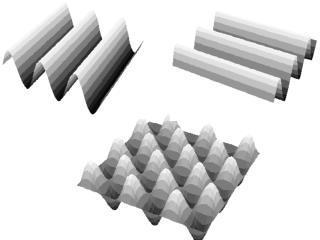Download PresentationR 1

# R 1 - PowerPoint PPT PresentationDownload Presentation## R 1

- - - - - - - - - - - - - - - - - - - - - - - - - - - E N D - - - - - - - - - - - - - - - - - - - - - - - - - - -
##### Presentation Transcript

1. Synchronous (in phase) waves from two point sources travel different path lengths to reach point A. A Point A will experience complete destructive interference if, for some integer m, the path difference =R1-R2 is exactly equal to 1. m 2. (m+ ½) 3. (m+ 1/4) 4. 0 R1 R2

2. =R1-R2  d /d = sin  = d sin

3. Diffraction through a slit

4. Double-Slit Interference r1 y r2  d  L For Destructive Interference  = 1/2, 3/2,5/2, 7/2, … = (m + 1/2)  For Constructive Interference  = 1, 2, 3, 4, … = m  d sin  = (m + 1/2)  d sin  = m  Path differencebetween waves determines phase difference Ifis small (r1, r2 >d) then:  = d sin  mis an integer: m = 0, ± 1, ± 2, ...

5. E c B The magnetron of a microwave horn generates a horizontally varying B-field and oscillating vertical E-field. We found which of the aluminum gratings below let the microwaves pass? 1 2

6. E c B

7. Exposed to Neon laser light passing uninterrupted through a narrow pair of slits, point P is the location of a bright fringe in the resulting interference pattern. P If a thin plate of clear crown glass held normal to the path to P covered one of the slits, the point P would • still be a point of constructive interference. • be a node (dark fringe) of destructive interference. • have a phase difference between the two slits that • depended on the thickness of the plate.

8. Exposed to Neon laser light passing uninterrupted through a narrow pair of slits, point P is the location of a bright fringe in the resulting interference pattern. P If a thin sheet of clear crown glass precision ground exactly to a thickness equal to an odd number of wavelengths N is held normal to the path to P covered one of the slits, the point P would nglass=1.5 • still be a point of constructive interference. • become a node (dark fringe) of destructive interference. • have a phase difference between the two slits that • depended on the number N.

9. A train of waves of fixed frequency is traveling along a thin, light cord toward the juncture to a heavy, thick cord. Both cords are stretched taut and under the same tension. The waves that travel across the heavy cord will have 1) greaterspeed than along the lighter cord.2) the same speed as along the lighter cord. 3) smaller speed than along the lighter cord.

10. A train of waves of fixed frequency is traveling along a thin, light cord toward the juncture to a heavy, thick cord. Both cords are stretched taut and under the same tension. The waves traveling across the heavy cord have 1) largerwavelength than along the lighter cord.2) the same wavelength as along the lighter cord. 3) smaller wavelength than along the lighter cord.

11. Another way to get Interference • Thin oil film on water: • Part of the incoming light is reflected off the top surface (point A), part at the lower surface (point B). • Light traveling through oil travels extra distance (from A to B to C). • If this distance is l, 2l, 3l, 4l, … • constructive interference! • If this distance is l/2, 3/2l, 5/2l, … • destructive interference!

12. A glass (n=1.60) lens is coated with a thin film of fluoride (MgF2, n=1.38). What minimum film thickness makes =550nm light non-reflecting?

13. A glass (n=1.60) lens is coated with a thin film of fluoride (MgF2, n=1.38). What minimum film thickness makes =550nm light non-reflecting? t=/4 180o phase change for each reflection